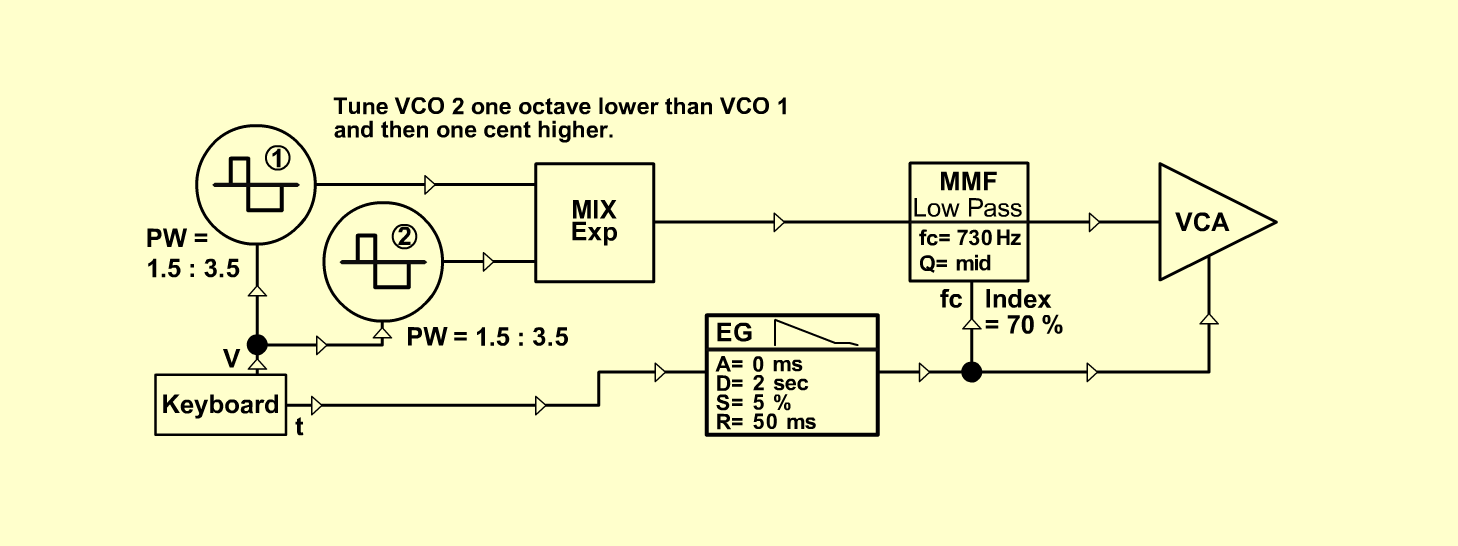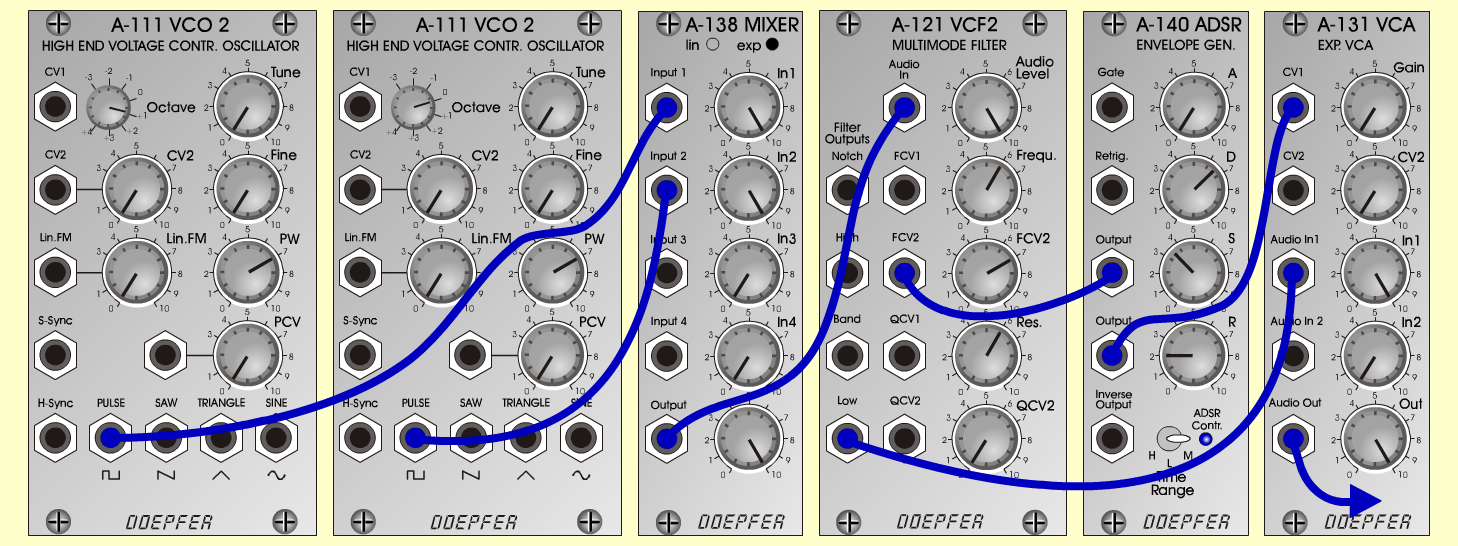NH-HarpsichordKeyboardsD o e p f e r A - 100 Connections: Settings: A-111/1 (Saw)  <=>  A-138exp (Input 1) A-111/2 (Saw)  <=>  A-138exp (Input 2) A-138exp (Output)  <=>  A-121 (Audio In) A-121 (Low)  <=>  A-131 (Audio In) A-140 (Output)  <=>  A-121 (FCV 2) A-140 (Output)  <=>  A-131 (CV 1) KB (CV)  <=>  A-111/1+2 (CV1) KB (Gate)  <=>  A-140 (Gate)  A-131 (Audio Out)  <=>  Amplifier A-111/2 (tune one octave lower than A-111/1 and then one cent higher) A-111/1 (PW = 7) A-111/2 (PW = 7) A-138 (In 1 = 10, In 2 = 10, Out = 10) A-121 (Audio Level = 10, Freq = 6, FCV 2 = 7, Res = 6)A-140 (A = 0, D = 6.5, S = 3.5, R = 2, Range = M) A-131 (Gain = 0, Audio In 1 = 10,  Audio Out = 10) Notes: Idea from Normann Hillesheim (Soundforum in the german magazin KEYBOARDS) Josef MuellerSound samples Bach: Chromatic Fantasia and Fugue (BWV 903) A Bach: Chromatic Fantasia and Fugue (BWV 903) B NH-Harpsichord (130 Hz) NH-Harpsichord (261 Hz) NH-Harpsichord (523 Hz)